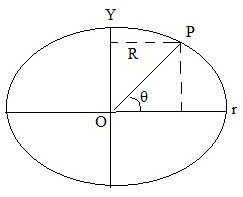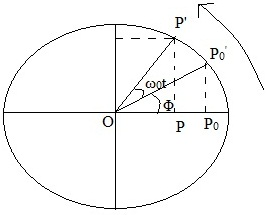#### Superposition of Harmonic Oscillations, Physics tutorial

Superposition of Simple Harmonic Oscillations:

When we superpose initial conditions corresponding to velocities and amplitudes, resultant displacement of two (or more) harmonic displacements will be algebraic sum of individual displacements at all subsequent times. The principle of superposition holds for any number of simple harmonic oscillations. These may be in same or mutually perpendicular directions, i.e. in two dimensions.

Given equation defines SHM:

d2x/dt2 = -ω02x........................................Eq.1

This is the linear homogeneous equation of second order. Such equation has significant property that sum of its two linearly independent solutions is itself a solution.

Let x1(t) and x2(t) respectively satisfy equations

d2x1/dt2 = -ω02x1........................................Eq.2

d2x2/dt2 = -ω02x2........................................Eq.3

Then by adding Eq.2 and Eq.3, we get

d2(x1 + x2)/dt2 = -ω02(x1 + x2) ........................................Eq.4

According to principle of superposition, sum of two displacements provided by

x(t) = x1(t) + x2(t) ........................................Eq.5

This also satisfies Eq.1. In other words, superposition of two displacements satisfies same linear homogeneous differential equation that is satisfied individually by x1 and x2.

Superposition of two harmonic oscillations of the same frequency along the same line:

Let us superpose two collinear (along same line) harmonic oscillations of amplitudes a1 and a2 having frequency ω0 and phase difference of π. Displacements of the oscillations are provided by

x1 = a1cosω0t.......................Eq.6

And x2 = a2cos(ω0t + π)

= -a2cosω0t.......................Eq.7

According to principle of superposition, resultant displacement is provided by

x(t) = x1(t) + x2(t)

= a1cosω0t - a2cosω0t

= (a1 - a2)cosω0t.......................Eq.8

This represents the simple harmonic motion of amplitude (a1 - a2). In particular, if two amplitudes are equal, i.e. a1 = a2, resultant displacement will be zero at all times.

Superposition of two collinear harmonic oscillations of different frequencies

In numerous cases, we have to deal with superposition of two or more harmonic oscillations having different angular frequencies. The microphone diaphragm and human eardrums are simultaneously subjected to different vibrations. For ease, we shall first consider superposition of two harmonic oscillations having same amplitude a but somewhat different frequencies ω1 and ω2 such that ω1 > ω2:

x1 = acos(ω1t + Φ1)

x2 = acos(ω2t + Φ2)

Phase difference between the two harmonic vibrations is:

Φ = (ω1 - ω2)t + (Φ1 - Φ2)

The first term (ω1 - ω2)t changes continuously with time. But second term (Φ1 - Φ2) is constant in time and as such it doesn't play any important role here. Thus, we may assume that initial phase of two oscillations are zero. Then, two harmonic oscillations can be written as:

x1(t) = acosω1t

x2(t) = acosω2t................Eq.9

The superposition of two oscillations gives the resultant

x(t) = x1(t) + x2(t) = acos(ω1t + ω2t)

This equation can be rewritten as

x(t) = 2acos((ω1 - ω2)/2)tcos((ω1 + ω2)/2)t

This is the oscillatory motion with angular frequency

1 + ω2)/2 and amplitude 2acos((ω1 - ω2)/2)t

The average angular frequency

ωav = (ω1 + ω2)/2 and the modulated angular frequency

ωmod = (ω1 - ω2)/2

We find that amplitude amod(t) = 2acosωmodt differs with the frequency ωmod/2π = (ω1 - ω2)/4π

This also signifies that in one complete cycle modulated amplitude takes values of 2a, 0, -2a, 0 and 2a for ωmod t = 0, π/2, π, 3π/2 and 2π, respectively. Resultant oscillation can be written as

x(t) = amod(t)cosωavt

This equation looks like the equation of SHM. But this resemblance is misleading. Modulated amplitude and phase constant are respectively provided by:

amod(t) = [a12 + a22 + 2a1a2cos(2ωmodt)]1/2

And θmod = [(a1 - a2)sinωmodt/(a1 + a2cosωmodt)]

Superposition of many harmonic oscillations of same frequency Method of Vector Addition:

This method is based on fact that displacement of the harmonic oscillation is projection of the uniform circular motion on diameter of circle. Thus, it is significant to understand connection between SHM and uniform circular motion.

Uniform Circular Motion and SHM:

Let us assume that a particle moves in the circle with constant speed V. Radius vector joining centre of circle and position of particle on the circumference will rotate with the constant angular frequency. We take x -axis to be along the direction of radius vector at time t = 0. Then angle made by the radius vector with x -axis at any time t will be given byθ = length of arc/radius of the circle = Vt/R

The x - and y - components of position of particle at time t are

x = Rcosθ and y = Rsinθ

Therefore, dx/dt = -Rsinθdθ/dt

= -ω0Rsinθ

As dθ/dt = ω0= V/R

Similarly, you can write

dy/dt = ω0Rcosθ

Differentiating again with respect to time, we get

d2x/dt2 = -ω02x and d2y/dt2 = -ω02y

These expressions show that when the particle moves uniformly in the circle, its projections along x - and y - axes execute SHM. A simple harmonic motion may be viewed as the projection of uniformly rotating vector on reference axis.

Assume that vector OP' with |OP'| = a0 is rotating with angular frequency ω0 in anticlockwise direction, as shown in Figure given below. Let P be foot of perpendicular drawn from P' on x - axis. Then OP = x is projection of OP' on x -axis. As vector OP' rotates at constant speed, point P executes simple harmonic motion along x -axis. Its period of oscillation is equivalent to period of rotating vector OP'. Let OP0' be initial position of the rotating vector. Its projection OP0 on x -axis is a0cosΦ. If rotating vector moves from OP0' to OP' in time t, then ∠P'OP0' ω0t and ∠P'O = (ω0t + Φ) Then we can writeOP = OP'cos∠P'Ox

or x = a0cos(ω0t + Φ)

Therefore, point P executes simple harmonic motion along x-axis. If you project OP' on y -axis, you will find that point corresponding to the foot of the normal satisfies equation

y = a0sin(ω0t + Φ)

This signifies that rotating vector can, in general, be resolved in two orthogonal components, and we can write

r = xx + yy

Where xx and yy are unit vectors along x - and y -axes, respectively.

Let us now consider superposition of n harmonic oscillations, all having same amplitude a0 and angular frequency ω0. Initial phases of successive oscillations differ by Φ0. Let first of these oscillations be defined by equation

x1(t) = a0cosω0t

Then, other oscillations are provided by:

x2(t) = a0cos(ω0t + Φ0).....xn(t) = a0cos[ω0t + (n-1)Φ0]

From principle of superposition, resultant oscillation is expressed by

x(t) = a0[cosω0t + cos(ω0t + Φ) + cos(ω0t + 2Φ0) +......cos(ω0t + (n-1)Φ0)]

Oscillations in two dimensions:

Oscillatory motion in two dimensions is also possible. Most recognizable example is motion of the simple pendulum whose bob is free to swing in any direction in x-y plane. This arrangement is known as spherical pendulum. We displace pendulum in x-direction and as we release it, we provide it impulse in the y-direction. The result is a composite motion when such a pendulum oscillates whose maximum x-displacement happens when y-displacement is zero and y velocity is maximum and vice versa. Remember that as the time period of the pendulum depends only on acceleration because of gravity and length of the cord, frequency of the superposed SHM's will be the same. Result is the curved path, in general, an ellipse. We now apply the principle of superposition to the case where two harmonic oscillations are perpendicular to each other.

Superposition of Two Mutually Perpendicular Harmonic Oscillations of Same Frequency:

Let two mutually perpendicular oscillations having amplitudes a1 and a2, such that a1 > a2 and angular frequency ω0. These are explained by equations

x1 = a1cosω0t and x2 = a2cos(ω0t + Φ)

Here we have taken initial stage of vibrations along x and the y-axes to be zero and Φ respectively. That is, Φ is a phase difference between two vibrations. First determine resultant oscillation for few particular values of phase difference

Case 1: Φ  = 0 or π

For Φ = 0 x = a1cosω0t and y = a2cosω0t

Therefore y/x = a2/a1 or y = (a2/a1)x

Likewise for Φ = π x = a1cosω0t and y = -a2cosω0t

So that y = -(a2/a1)x

The equation of y given above explains straight lines passing through origin. This signifies that resultant motion of particle is along straight line. Though for Φ = 0 the motion is along one diagonal but when Φ = π the motion is along the other diagonal.

Case 2: Φ = π/2. In this case two vibrations are provided by

x = a1cosω0t

y = a2cos(ω0t + π/2) = -a2sinω0t

On squaring the expressions and adding resultant expressions, we get

x2/a12 + y2/a22 = cos2Φ + sin2Φ = 1

This is equation of ellipse. Therefore resultant motion of particle is along ellipse whose principal axes lie along x- and y-axes. Semi-major and semi-minor axes of ellipse are a1 and a2. As time increases x decreases from maximum positive value but y becomes more and more negative. Therefore, ellipse is explained in clockwise direction. If you analyze case when Φ = 3π/2 or Φ = π/2, you will get same ellipse, but motion will be in anticlockwise direction.

When amplitudes a1 and a2 are equal, a1 = a2 = a Equation given above reduces to

x2 + y2 = a2

This equation represents the circle of radius a. This signifies that ellipse reduces to circle. This equation represents the circle of radius a. This denotes that ellipse reduces to the circle.

Tutorsglobe: A way to secure high grade in your curriculum (Online Tutoring)

Expand your confidence, grow study skills and improve your grades.

Since 2009, Tutorsglobe has proactively helped millions of students to get better grades in school, college or university and score well in competitive tests with live, one-on-one online tutoring.

Using an advanced developed tutoring system providing little or no wait time, the students are connected on-demand with a tutor at www.tutorsglobe.com. Students work one-on-one, in real-time with a tutor, communicating and studying using a virtual whiteboard technology.  Scientific and mathematical notation, symbols, geometric figures, graphing and freehand drawing can be rendered quickly and easily in the advanced whiteboard.

Free to know our price and packages for online physics tutoring. Chat with us or submit request at info@tutorsglobe.com